Introduction to forest plots

2019-06-24

Forest plots date back to 1970s and are most frequently seen in meta-analysis, but are in no way restricted to these. The forestplot package is all about providing these in R. It originated form the ‘rmeta’-package’s forestplot function and has a part from generating a standard forest plot, a few interesting features:

• Text:
• Ability to use a table of text, i.e. the text can consist of several columns if needed‡
• Ability to use expressions within your text that permit mathematical symbols, e.g. expression(beta)
• Set the gpar arguments (fontfamily, fontface, cex, etc) for both summary and regular rows. This can be specified down to the each cell.
• Confidence intervals:
• Clip confidence intervals to arrows when they exceed specified limits‡
• Multiple confidence bands for the same row
• Choose between different estimate markers such as boxes, diamonds, points
• Custom confidence interval drawing functions
• Legends:
• Have a legend on top or to the left of the plot
• Have the legend within the plot’s graph section
• Put a box around legend (sharp or rounded corners)
• Other:
• Dividing the graph visually by adding horizontal lines
• Choose line height to either adapt to viewport (graph) size or specify an exact height in units
• Choose between a zero-effect line line or an area box
• Use flexible arguments, you can choose if you want to provide mean, lower, and upper separately or within one array.

‡ Features present int the original rmeta::forestplot function.

Note: An important difference from the original forestplot is that the current function interprets xlog as the x-axis being in log-format, i.e. you need to provide the data in the antilog/exp format.

Text

A forest plot is closely connected to text and the ability to customize the text is central.

Table of text

Below is a basic example from the original forestplot function that shows how to use a table of text: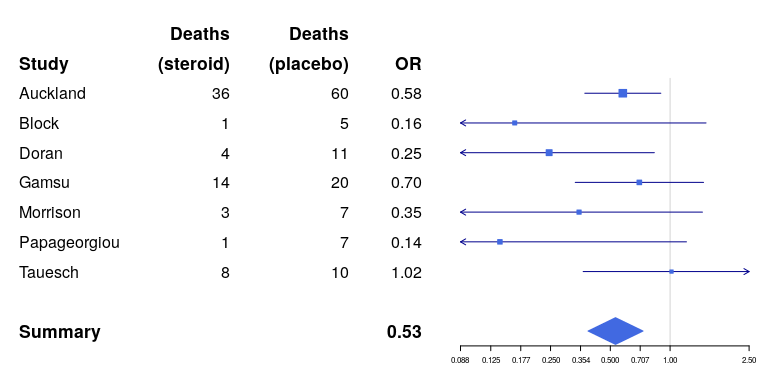Summary lines

The same as above but with lines based on the summary elements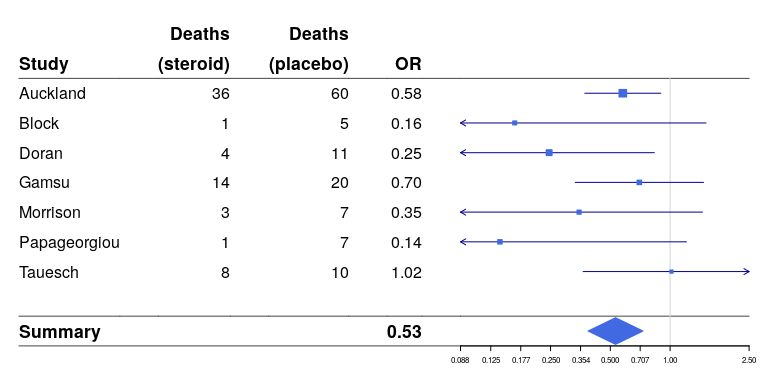We can also choose what lines we want by provifing a list where the name is the line number affected, in the example below 3rd line and 11th counting the first line to be above the first row (not that there is an empty row before summary):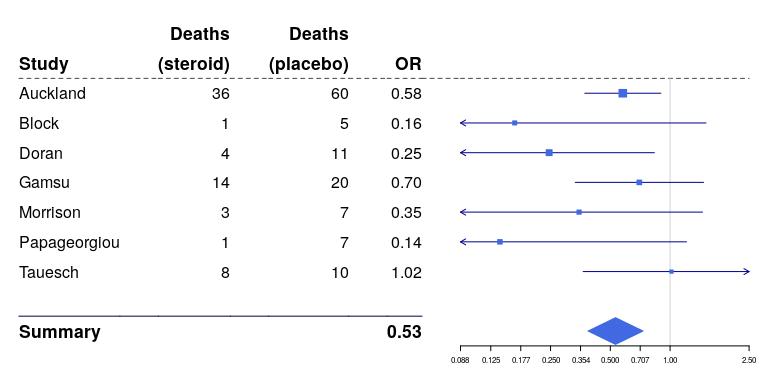For marking the start/end points it is common to add a vertical line at the end of each whisker. In forestplot you simply specify the vertices argument: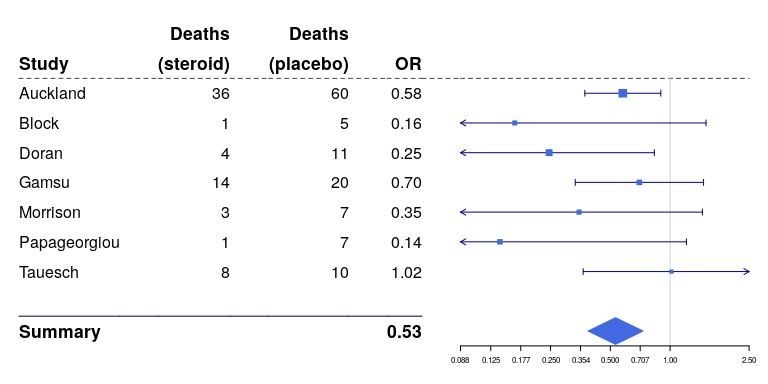Positioning the graph element

You can also choose to have the graph positioned within the text table by specifying the graph.pos argument: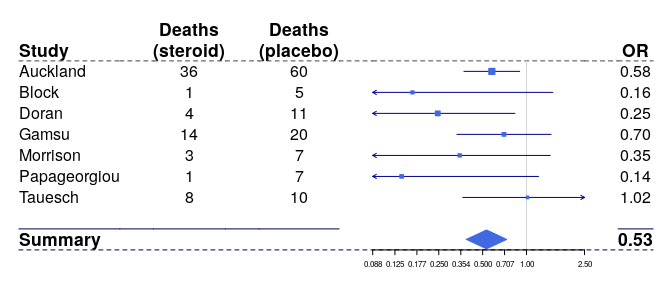Using expressions

If we present a regression output it is sometimes convenient to have non-ascii letters. We will use my study comparing health related quality of life 1 year after total hip arthroplasties between Sweden and Denmark for this section: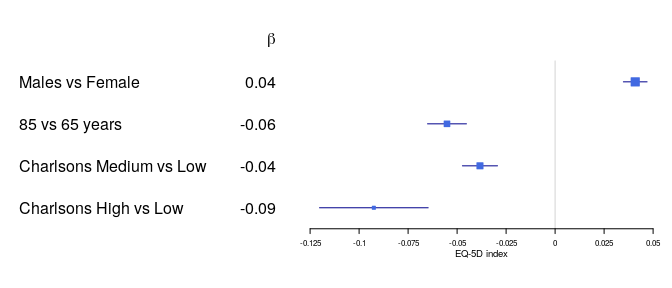Altering fonts

Altering fonts may give a completely different feel to the table: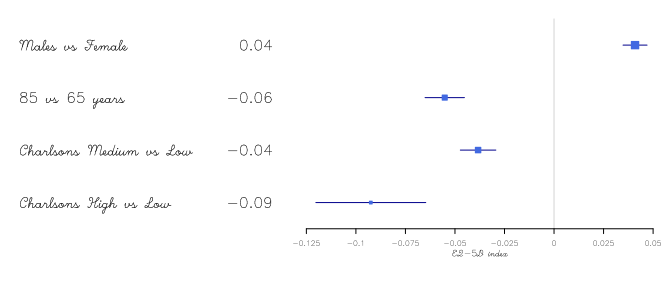There is also the possibility of being selective in gp-styles: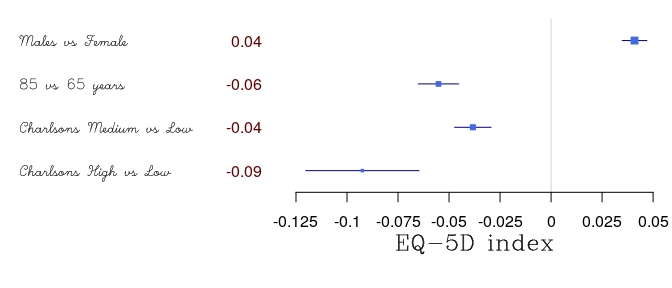Confidence intervals

Clipping the interval is convenient for uncertain estimates in order to retain the resolution for those of more interest. The clipping simply adds an arrow to the confidence interval, see the bottom estimate below:

forestplot(tabletext,
rbind(HRQoL$Sweden), clip =c(-.1, Inf), col=clrs, xlab="EQ-5D index")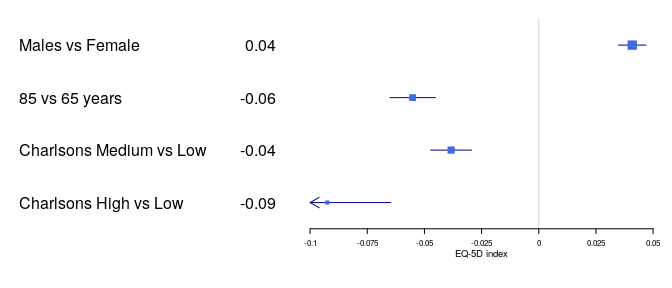Multiple confidence bands When combining similar outcomes for the same exposure I’ve found it useful to use multiple bands per row. This efficiently increases the data-ink ratio while making the comparison between the two bands trivial. The first time I’ve used this was in my article comparing Swedish with Danish patients 1 year after total hip arthroplasty. Here the clipping also becomes obvious as the Danish sample was much smaller, resulting in wider confidence intervals.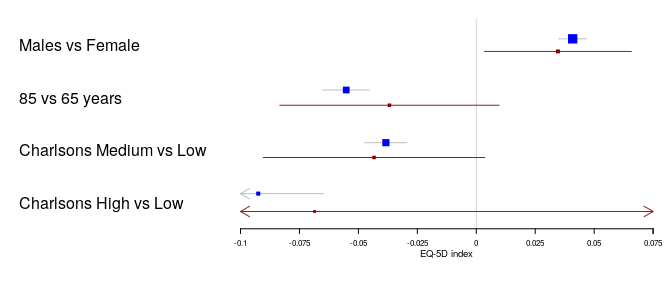Estimate indicator You can choose between a number of different estimate indicators. Using the example above we can set the Danish results to circles.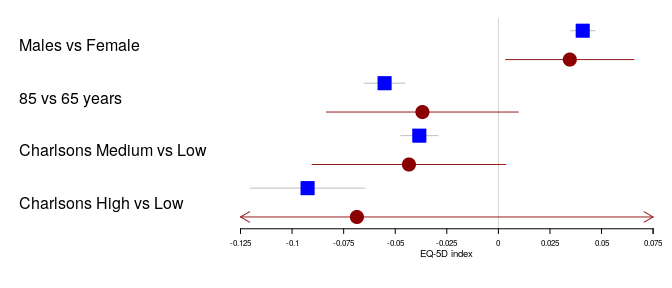The confidence interval/box drawing functions are fully customizeable. You can write your own function that accepts the parameters: lower_limit, estimate, upper_limit, size, y.offset, clr.line, clr.marker, and lwd. Choosing line type You can furthermore choose between all available line types through the lty.ci that can also be specified element specific.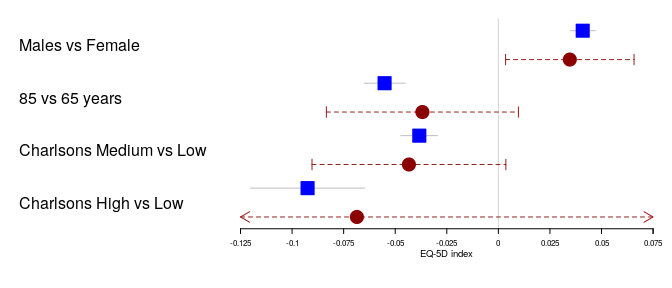Legends Adding a basic legend is done through the legend argument: forestplot(tabletext, legend = c("Sweden", "Denmark"), fn.ci_norm = c(fpDrawNormalCI, fpDrawCircleCI), boxsize = .25, # We set the box size to better visualize the type line.margin = .1, # We need to add this to avoid crowding mean = cbind(HRQoL$Sweden[, "coef"], HRQoL$Denmark[, "coef"]), lower = cbind(HRQoL$Sweden[, "lower"], HRQoL$Denmark[, "lower"]), upper = cbind(HRQoL$Sweden[, "upper"], HRQoL$Denmark[, "upper"]), clip =c(-.125, 0.075), col=fpColors(box=c("blue", "darkred")), xlab="EQ-5D index")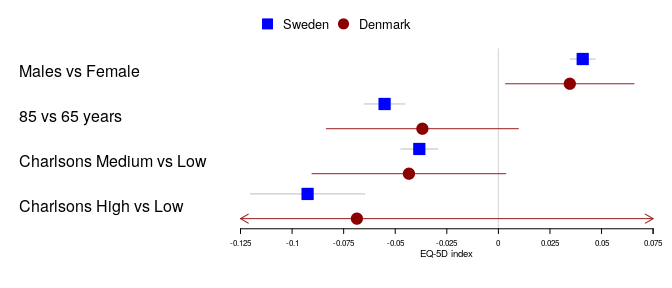This can be further customized by setting the legend_args argument using the fpLegend function: forestplot(tabletext, legend_args = fpLegend(pos = list(x=.85, y=0.25), gp=gpar(col="#CCCCCC", fill="#F9F9F9")), legend = c("Sweden", "Denmark"), fn.ci_norm = c(fpDrawNormalCI, fpDrawCircleCI), boxsize = .25, # We set the box size to better visualize the type line.margin = .1, # We need to add this to avoid crowding mean = cbind(HRQoL$Sweden[, "coef"], HRQoL$Denmark[, "coef"]), lower = cbind(HRQoL$Sweden[, "lower"], HRQoL$Denmark[, "lower"]), upper = cbind(HRQoL$Sweden[, "upper"], HRQoL$Denmark[, "upper"]), clip =c(-.125, 0.075), col=fpColors(box=c("blue", "darkred")), xlab="EQ-5D index")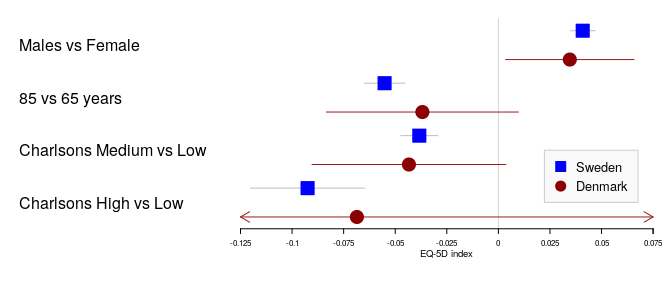Ticks and grids If the automated ticks don’t match the desired once it is easy to change these using the xticks argument: forestplot(tabletext, legend = c("Sweden", "Denmark"), fn.ci_norm = c(fpDrawNormalCI, fpDrawCircleCI), boxsize = .25, # We set the box size to better visualize the type line.margin = .1, # We need to add this to avoid crowding mean = cbind(HRQoL$Sweden[, "coef"], HRQoL$Denmark[, "coef"]), lower = cbind(HRQoL$Sweden[, "lower"], HRQoL$Denmark[, "lower"]), upper = cbind(HRQoL$Sweden[, "upper"], HRQoL$Denmark[, "upper"]), clip =c(-.125, 0.075), col=fpColors(box=c("blue", "darkred")), xticks = c(-.1, -0.05, 0, .05), xlab="EQ-5D index")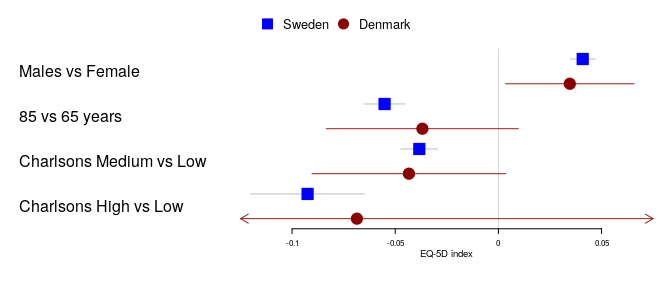By adding a “labels” attribute to the ticks you can tailor the ticks even further, here’s an example the supresses tick text for every other tick: xticks <- seq(from = -.1, to = .05, by = 0.025) xtlab <- rep(c(TRUE, FALSE), length.out = length(xticks)) attr(xticks, "labels") <- xtlab forestplot(tabletext, legend = c("Sweden", "Denmark"), fn.ci_norm = c(fpDrawNormalCI, fpDrawCircleCI), boxsize = .25, # We set the box size to better visualize the type line.margin = .1, # We need to add this to avoid crowding mean = cbind(HRQoL$Sweden[, "coef"], HRQoL$Denmark[, "coef"]), lower = cbind(HRQoL$Sweden[, "lower"], HRQoL$Denmark[, "lower"]), upper = cbind(HRQoL$Sweden[, "upper"], HRQoL$Denmark[, "upper"]), clip =c(-.125, 0.075), col=fpColors(box=c("blue", "darkred")), xticks = xticks, xlab="EQ-5D index")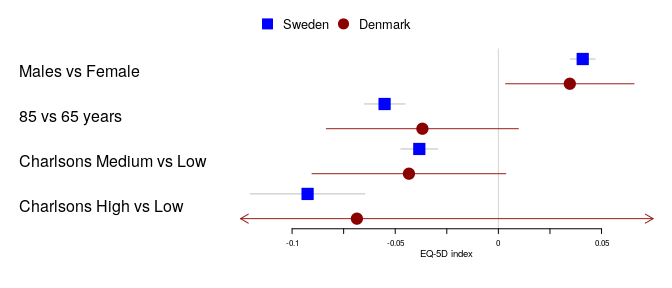Sometimes you have a very tall graph and you want to add helper lines in order to make it easier to see the tick marks. This can be useful in non-inferiority or equivalence studies. You can do this through the grid argument: forestplot(tabletext, legend = c("Sweden", "Denmark"), fn.ci_norm = c(fpDrawNormalCI, fpDrawCircleCI), boxsize = .25, # We set the box size to better visualize the type line.margin = .1, # We need to add this to avoid crowding mean = cbind(HRQoL$Sweden[, "coef"], HRQoL$Denmark[, "coef"]), lower = cbind(HRQoL$Sweden[, "lower"], HRQoL$Denmark[, "lower"]), upper = cbind(HRQoL$Sweden[, "upper"], HRQoL$Denmark[, "upper"]), clip =c(-.125, 0.075), col=fpColors(box=c("blue", "darkred")), grid = TRUE, xticks = c(-.1, -0.05, 0, .05), xlab="EQ-5D index")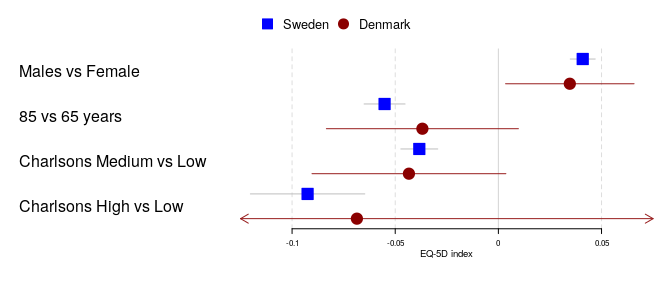You can easily customize both what grid lines to use and what type they should be by adding the gpar object to a vector: forestplot(tabletext, legend = c("Sweden", "Denmark"), fn.ci_norm = c(fpDrawNormalCI, fpDrawCircleCI), boxsize = .25, # We set the box size to better visualize the type line.margin = .1, # We need to add this to avoid crowding mean = cbind(HRQoL$Sweden[, "coef"], HRQoL$Denmark[, "coef"]), lower = cbind(HRQoL$Sweden[, "lower"], HRQoL$Denmark[, "lower"]), upper = cbind(HRQoL$Sweden[, "upper"], HRQoL\$Denmark[, "upper"]),
clip =c(-.125, 0.075),
col=fpColors(box=c("blue", "darkred")),
grid = structure(c(-.1, -.05, .05),
gp = gpar(lty = 2, col = "#CCCCFF")),
xlab="EQ-5D index")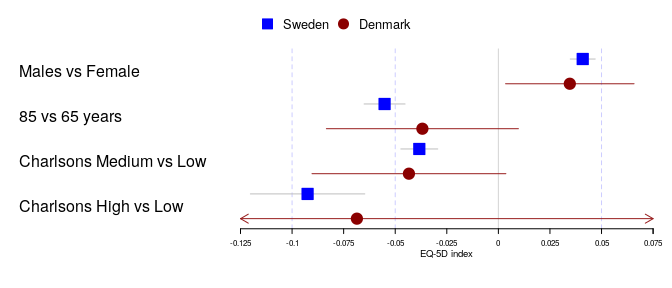If you are unfamiliar with the structure call it is equivalent to generating a vector and then setting an attribute, eg:

grid_arg <- c(-.1, -.05, .05)
attr(grid_arg, "gp") <- gpar(lty = 2, col = "#CCCCFF")

identical(grid_arg,
structure(c(-.1, -.05, .05),
gp = gpar(lty = 2, col = "#CCCCFF")))
# Returns TRUE

Ok, that’s it. I hope you find the package forestplot useful.